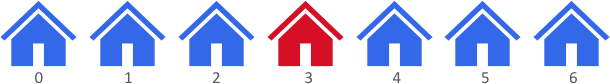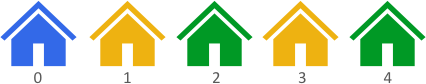2078. Two Furthest Houses With Different Colors

#### QUESTION:

There are n houses evenly lined up on the street, and each house is beautifully painted. You are given a 0-indexed integer array colors of length n, where colors[i] represents the color of the ith house.

Return the maximum distance between two houses with different colors.

The distance between the ith and jth houses is abs(i - j), where abs(x) is the absolute value of x.

Example 1:``````Input: colors = [1,1,1,6,1,1,1]
Output: 3
Explanation: In the above image, color 1 is blue, and color 6 is red.
The furthest two houses with different colors are house 0 and house 3.
House 0 has color 1, and house 3 has color 6. The distance between them is abs(0 - 3) = 3.
Note that houses 3 and 6 can also produce the optimal answer.
``````

Example 2:``````Input: colors = [1,8,3,8,3]
Output: 4
Explanation: In the above image, color 1 is blue, color 8 is yellow, and color 3 is green.
The furthest two houses with different colors are house 0 and house 4.
House 0 has color 1, and house 4 has color 3. The distance between them is abs(0 - 4) = 4.
``````

Example 3:

``````Input: colors = [0,1]
Output: 1
Explanation: The furthest two houses with different colors are house 0 and house 1.
House 0 has color 0, and house 1 has color 1. The distance between them is abs(0 - 1) = 1.
``````

Constraints:

``````n == colors.length
2 <= n <= 100
0 <= colors[i] <= 100
Test data are generated such that at least two houses have different colors.
``````

#### EXPLANATION:

easy的题目, 一个for循环就可以搞定了.

#### SOLUTION:

``````class Solution {
func maxDistance(_ colors: [Int]) -> Int {
var result:Int = 0
for indexI in 0...colors.count - 2 {
for indexJ in indexI + 1...colors.count - 1 {
if colors[indexI] != colors[indexJ] {
result = max(result, abs(indexJ - indexI))
}
}
}
return result
}
}
``````